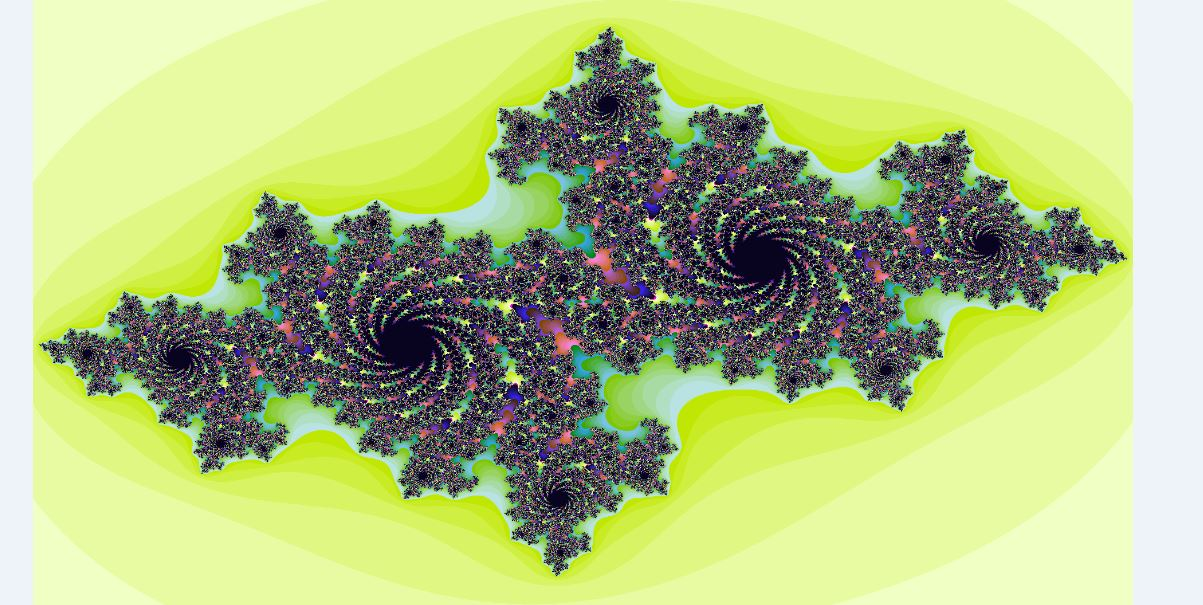Related Articles

# Julia fractal in Python

• Last Updated : 03 Oct, 2018

Introduction to julia set
In the context of complex dynamics, a topic of mathematics, the Julia set and the Fatou set are two complementary sets (Julia ‘laces’ and Fatou ‘dusts’) defined from a function. Informally, the Fatou set of the function consists of values with the property that all nearby values behave similarly under repeated iteration of the function, and the Julia set consists of values such that an arbitrarily small perturbation can cause drastic changes in the sequence of iterated function values. Thus the behavior of the function on the Fatou set is ‘regular’, while on the Julia set its behavior is ‘chaotic’.
The Julia set of a function f is commonly denoted J(f), and the Fatou set is denoted F(f). These sets are named after the French mathematicians Gaston Julia and Pierre Fatou whose work began the study of complex dynamics during the early 20th century. [Source Wiki]

The equation to generate Julia fractal is:where c is a complex parameter. The Julia set for this system is the subset of the complex plane given by:So let’s now try to create one of the fractal in the above image.

To do so we need the Pillow module of python which makes it easy to dealt with images and stuff.

To install pillow through pip type the following command in the command prompt.

pip install Pillow

Now using this library to create the fractal image.

 # Python code for Julia Fractalfrom PIL import Image   # driver functionif __name__ == "__main__":        # setting the width, height and zoom     # of the image to be created    w, h, zoom = 1920,1080,1       # creating the new image in RGB mode    bitmap = Image.new("RGB", (w, h), "white")      # Allocating the storage for the image and    # loading the pixel data.    pix = bitmap.load()         # setting up the variables according to     # the equation to  create the fractal    cX, cY = -0.7, 0.27015    moveX, moveY = 0.0, 0.0    maxIter = 255       for x in range(w):        for y in range(h):            zx = 1.5*(x - w/2)/(0.5*zoom*w) + moveX            zy = 1.0*(y - h/2)/(0.5*zoom*h) + moveY            i = maxIter            while zx*zx + zy*zy < 4 and i > 1:                tmp = zx*zx - zy*zy + cX                zy,zx = 2.0*zx*zy + cY, tmp                i -= 1              # convert byte to RGB (3 bytes), kinda             # magic to get nice colors            pix[x,y] = (i << 21) + (i << 10) + i*8      # to display the created fractal    bitmap.show()

Output:After understanding the code try to draw the other fractals by changing the value of the variables and post your github link to the code down in the comment section and i’ll be happy to help you if any error comes up.

This article is contributed by Subhajit Saha. If you like GeeksforGeeks and would like to contribute, you can also write an article using contribute.geeksforgeeks.org or mail your article to contribute@geeksforgeeks.org. See your article appearing on the GeeksforGeeks main page and help other Geeks.